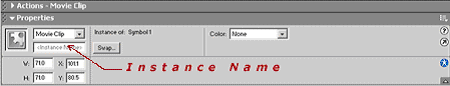# Flash Script – Scrolling Menu With A Scrolling Background

This is a popular effect, and it’s easy to do! Here’s how…

1. Set the frame properties first. The size should be 420 X 90 pixels, and the frame rate should be 25 frames per second.

2. Import a background picture. I made the background picture in photoshop, and its size was 700 X 85 pixels.

3. Select the background picture and convert it to a movie clip. Name it bg_static.

4. Give this an instance name of "bg".5. Create a movieclip that’s 1045 X 40 pixels. Give this an instance name of "menu".

6. Select the first frame and insert this action:

``````xm = 0;
//function to set the xpos of the movieclip
function xpos(bar_length,mul)
{
hpos = 0;
scroll_length = 420;
incr = bar_length/scroll_length;
xm = _xmouse;
if(_xmouse <= 10){xm = 10;}
if(_xmouse >= 400){xm = 400;}
scroll_x = hpos - xm;
scroll_x = scroll_x * mul;
x_pos = scroll_x * incr;
x_pos = x_pos + hpos;
return x_pos;
}

_root.onEnterFrame = function ()
{
// call function xpos
x_pos = xpos(700,.20);
with (bg)
{
_x += (x_pos - _x)*.4;
}
// call function xpos
x_pos = xpos(950,.75);